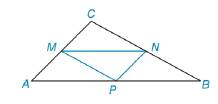Chapter 8.1, Problem 33E### Elementary Geometry for College St...

6th Edition
Daniel C. Alexander + 1 other
ISBN: 9781285195698

#### Solutions

Chapter
Section### Elementary Geometry for College St...

6th Edition
Daniel C. Alexander + 1 other
ISBN: 9781285195698
Textbook Problem
1 views

# Given △ A B C with midpoints M, N and P of the sides, explain why A A B C = 4 ⋅ A M N P .To determine

To find:

AABC=4AMNP. In ABC with midpoint M, N and P of the sides,

Explanation

Given,

ABC with midpoints M, N and P of the sides,

Let AB=a

BC=b and AC=c

P is the midpoint of AB,

Then AP=a2, and PB=a2

N is the midpoint of BC.

Then, BN=b2, and NC=b2

M is the midpoint of AC,

Then, AM=c2, and MC=c2

Thus, MNPB, NPAM, and MPNC

(i.e.) MN=PB=a2

NP=AM=c2

MP=CN=b2

Now, the figure becomes,

From the figure, the dimensions of inscribed triabngles MNP, AMP, PNB, and NCM are same

### Still sussing out bartleby?

Check out a sample textbook solution.

See a sample solution

#### The Solution to Your Study Problems

Bartleby provides explanations to thousands of textbook problems written by our experts, many with advanced degrees!

Get Started

#### If H() = sin , find H'() and H"( ).

Single Variable Calculus: Early Transcendentals, Volume I

#### If 0 a b, find limt0{01[bx+a(1x)]tdx}1/t

Single Variable Calculus: Early Transcendentals

#### Proof prove that cos(sin1x)=1x2

Calculus: Early Transcendental Functions (MindTap Course List)

#### Evaluate .

Study Guide for Stewart's Single Variable Calculus: Early Transcendentals, 8th